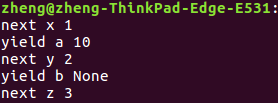淺談Python協程中的yield表示式

python生成器

python中生成器是迭代器的一種，使用yield返回函式值。每次呼叫yield會暫停，而可以使用next()函式和send()函式可以恢復生成器。

注意到yield是個表示式而不僅僅是個語句，所以可以使用x = yield r 這樣的語法。

python使用生成器來實現協程，下面看一個python協程應用於生產者消費者問題的例子

def consumer():
r = 'yield'
while True:
#當下邊語句執行時，先執行yield r，然後consumer暫停，此時賦值運算還未進行
#等到producer呼叫send()時，send()的引數作為yield r表示式的值賦給等號左邊
n = yield r #yield表示式可以接收send()發出的引數
if not n:
return
print('[CONSUMER] Consuming %s...' % n)
r = '200 OK'

def produce(c):
c.send(None)
n = 0
while n < 5:
n = n + 1
print('[PRODUCER] Producing %s...' % n)
r = c.send(n)   #呼叫consumer生成器
print('[PRODUCER] Consumer return: %s' % r)
c.close()

c = consumer()
produce(c)

def gen():
a = yield 1
print('yield a % s' % a)
b = yield 2
print('yield b % s' % b)
c = yield 3
print('yield c % s' % c)

r = gen()
x = next(r)
print('next x %s' % x)
y = r.send(10)
print('next y %s' %y)
z = next(r)
print('next z %s' % z)